﻿ 船舶稳性控制系统研究
 舰船科学技术2022, Vol. 44Issue (10): 115-118    DOI: 10.3404/j.issn.1672-7649.2022.10.023PDF

1. 江苏海事职业技术学院，江苏 南京 211170;
2. 上海海事大学 商船学院，上海 200135

Research on ship stability control system
XIE Bao-feng1, XI Yong-tao2, ZHANG Shu-kui1, QU Fang1
1. Jiangsu Maritime Institute, Nanjing 211170, China;
2. Merchant Marine College Shanghai Maritime University, Shanghai 200135, China
Abstract: Stability control is the key link of ship navigation motion control. Because the ship will be affected by regular waves, irregular waves and other loads when sailing in seawater, when the motion of the ship under the external load exceeds the limit equilibrium position, it may lead to the instability of the ship and serious accidents such as ship overturning. Therefore, researchers in the field of ships regard stability control as an important research field. In this paper, the multi coordinate system and coordinate transformation of ship motion control process are established, and a ship stability control system is designed by using controllable anti rolling tank technology. The research is carried out from the composition principle of the control system and the simulation of the stability control system.
Key words: stability control     regular wave     anti rolling tank     overturning instability
0 引　言

1 船舶稳性运动坐标系及波浪载荷的建模

1）固定坐标系O-LMN，坐标系原点位于静止水面上，ON轴竖直向下，OL轴指向波浪正向，固定坐标系用来描述波浪；

2）运动坐标系O-XYZ，以船舶的重心G为坐标系原点，OX轴平行于甲板面指向船舷；

3）参考坐标系o-xyz；该坐标系以船舶的航行速度随船一起运动，描述船舶自身运动。图 1 船舶稳性运动坐标系 Fig. 1 Ship stability motion coordinate system

 $\begin{gathered} X = x ，\hfill \\ Y = y\cos \varphi - z\sin \varphi ，\hfill \\ Z = y\sin \varphi + z\cos \varphi ，\hfill \\ \end{gathered}$

 $\left[ {\begin{array}{*{20}{l}} X \\ Y \\ Z \end{array}} \right] = \left[ {\begin{array}{*{20}{c}} 1&0&0 \\ 0&{\cos \varphi }&{ - \sin \varphi } \\ 0&{\sin \varphi }&{\cos \varphi } \end{array}} \right]\left[ {\begin{array}{*{20}{l}} x \\ y \\ z \end{array}} \right] \text{，}$

 $\left[ {\begin{array}{*{20}{l}} l \\ m \\ n \end{array}} \right] = \left[ {\begin{array}{*{20}{l}} {\cos \theta \cos \beta }&{ - \cos \varphi \sin \beta }&{\cos \varphi } \\ {\cos \theta \sin \beta }&{\cos \varphi \cos \beta }&{\cos \beta } \\ { - \sin \theta }&{\sin \varphi \cos \theta }&{\cos \varphi \cos \theta } \end{array}} \right]\left[ {\begin{array}{*{20}{l}} x \\ y \\ z \end{array}} \right] \text{，}$

 $\left\{ {\begin{array}{*{20}{c}} {\left( {{J_0} + \Delta {J_{}}} \right)w + 2\kappa w + \dfrac{1}{2}Dh\theta = {T_0}} ，\\ {M\dfrac{{{\rm{d}}v}}{{{\rm{d}}t}}\sin \theta + M\dfrac{{{\rm{d}}u}}{{\rm{d}}t}\cos \theta = {F_0}} 。\end{array}} \right.$

 $t = \sqrt 2 \text{π} \sqrt {\frac{{v + \Delta v}}{{D\sin \theta }}} 。$

 $f\left( t \right) = {\theta _m} - B\sin \left( {{w_o}t + {\varphi _0}} \right) \text{。}$

 ${\theta _m} = \frac{{2\text{π}\zeta }}{\lambda } \text{。}$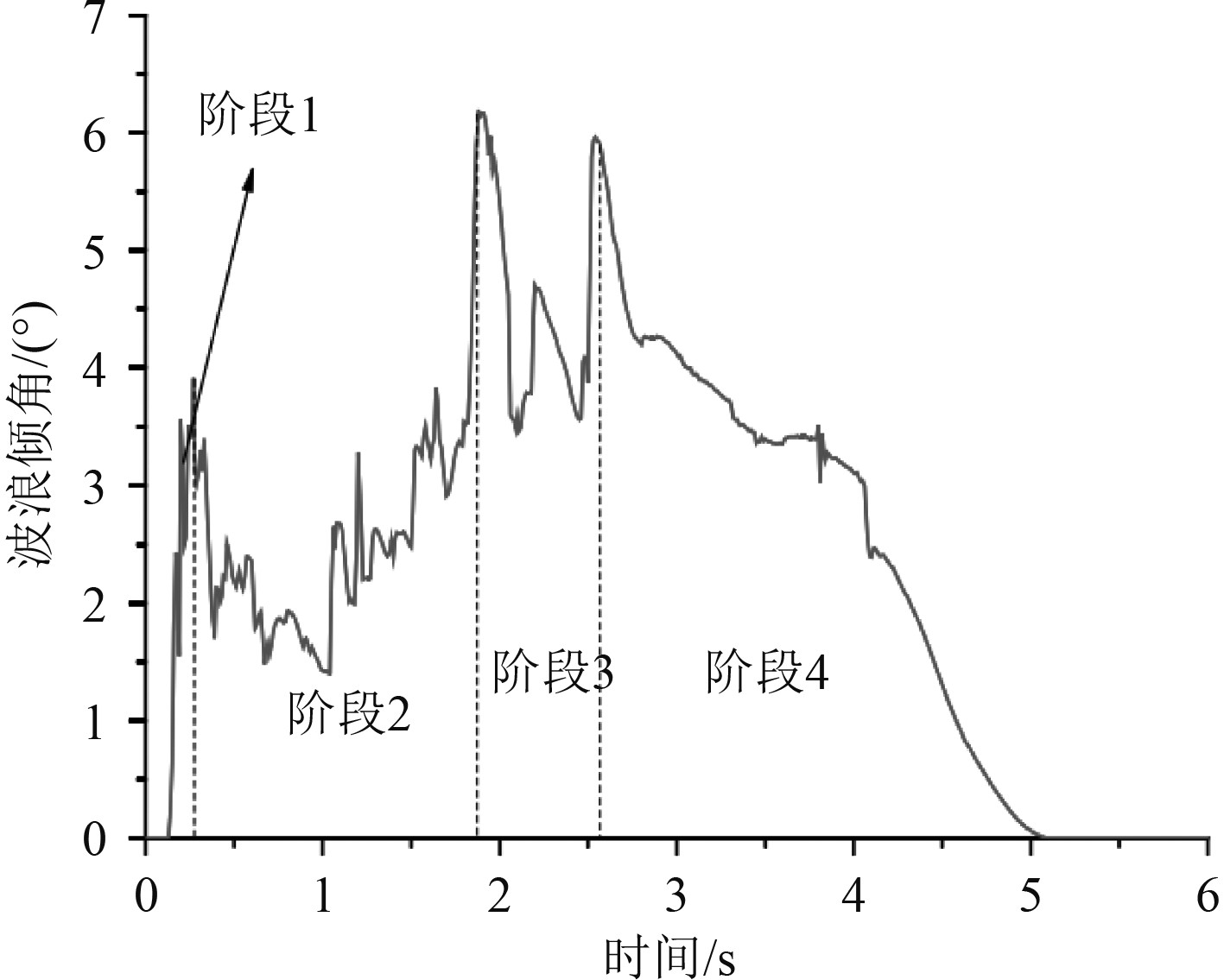图 2 0～5 s周期海浪的波面倾角曲线 Fig. 2 Wave surface inclination curve of wave in 0～5 s period
2 船舶稳性控制系统设计 2.1 船舶稳性控制原理介绍

1）增大阻尼系数

2）降低船体固有频率

3）降低扰动力或力矩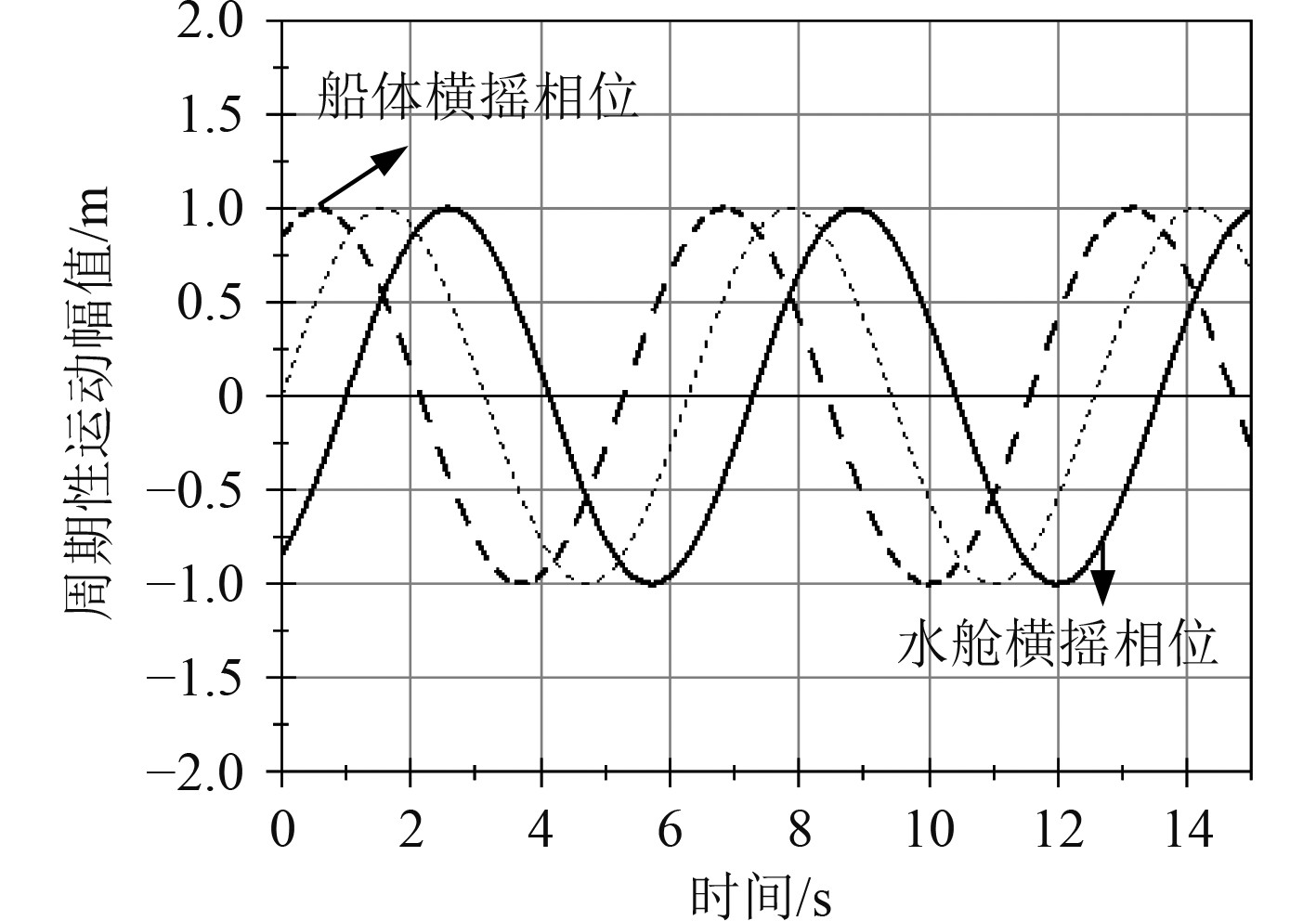图 3 船舶减摇水舱与扰动力矩的相位图 Fig. 3 Phase diagram of ship anti rolling tank and disturbance torque
2.2 船舶稳性控制系统硬件组成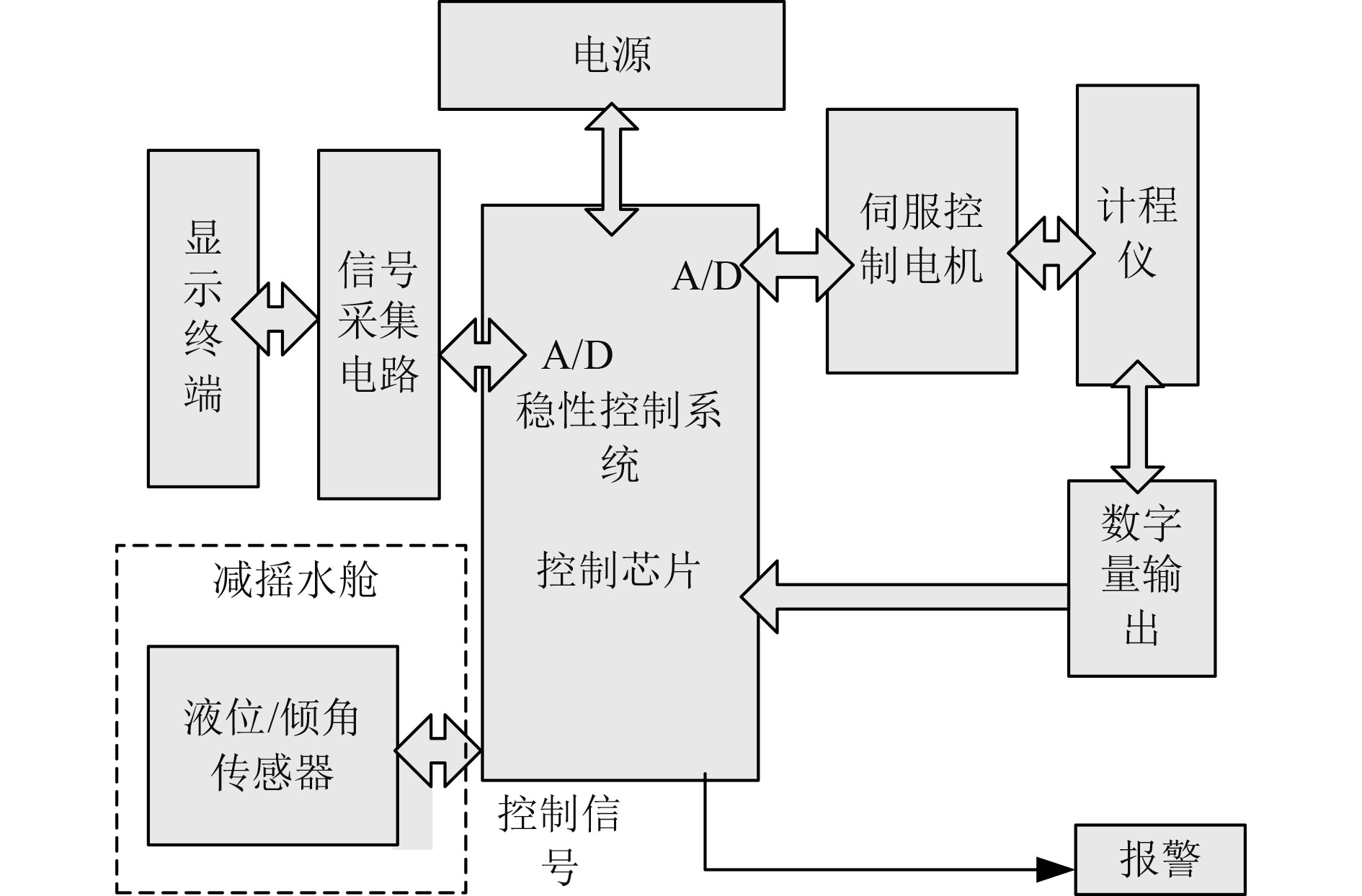图 4 船舶稳性控制系统的硬件原理图 Fig. 4 Hardware principle of ship stability control system

1）伺服驱动电机表 1 伺服驱动电机的参数表 Tab.1 Parameter table of servo motor

2）控制芯片

3）信号采集电路

1）远程控制功能

2）分布式控制

3）智能控制

3 船舶稳性控制系统仿真 3.1 稳性控制试验台设计

1）几何相似性

 $\delta=\frac{L_{H}}{L_{{K}}} \text{。}$

2）运动相似性

 ${\xi _h} = \frac{{{V_H}{T_H}}}{{{L_H}}} = \frac{{{V_K}{T_K}}}{{{L_K}}} \text{。}$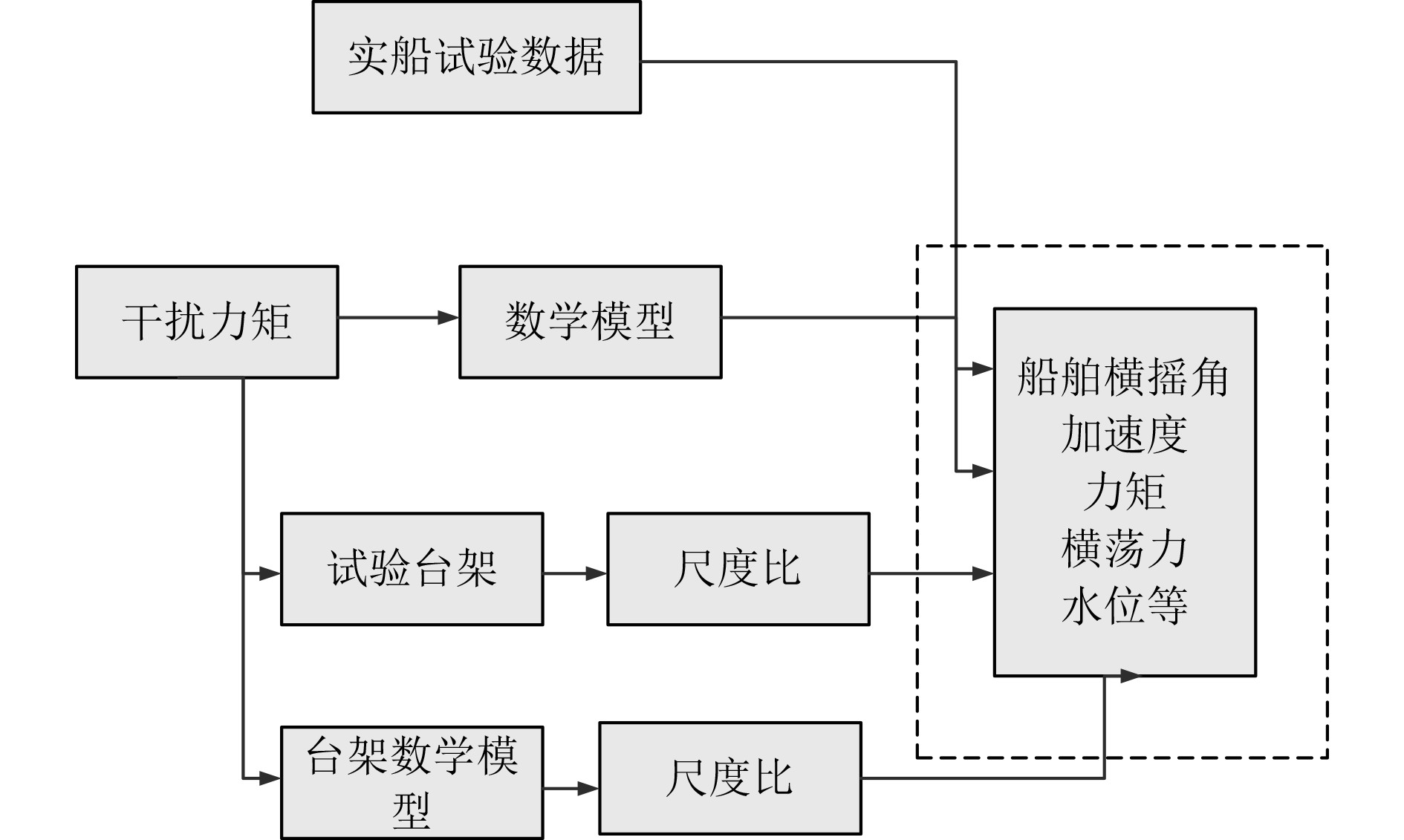图 5 仿真试验台的工作原理 Fig. 5 Working principle of simulation test-bed
3.2 船舶稳性控制仿真试验

 $f = \sqrt 2 \text{π} \sqrt {\frac{1}{{D\sin \theta }}} = 100\;{\rm{H}}{\rm{z}} \text{。}$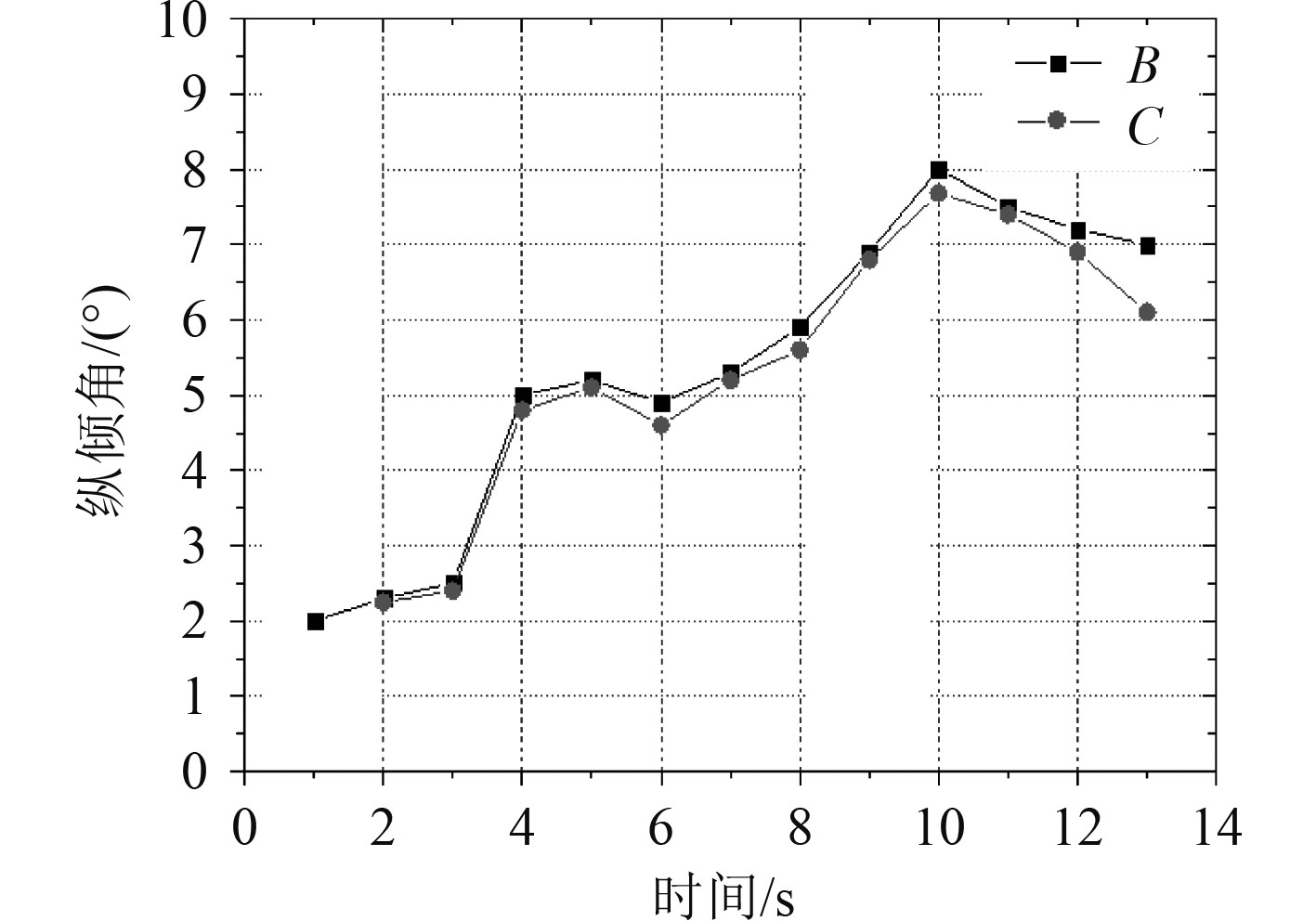图 6 输入激励与船舶实际横摇角度的关系曲线 Fig. 6 Relation curve between input excitation and actual rolling angle of ship
4 结　语

  耿东升, 唐友刚, 李红霞. 船舶海浪中非线性随机运动的可视化技术[J]. 计算机仿真, 2007, 24(3): 5.  耿东升, 唐友刚, 李红霞. 船舶海浪中非线性随机运动的可视化技术[J]. 计算机仿真, 2007, 24(3): 210-214. DOI:10.3969/j.issn.1006-9348.2007.03.056  范佘明, 盛子寅, 陶尧森, 等. 船舶在波浪中的操纵运动预报[J]. 中国造船, 2001(2): 28-35.  卜淑霞, 顾民, 黄苗苗. 波浪中破损船舶的运动响应研究[J]. 船舶力学, 2017(z1): 9.  李晨, 陈永冰, 李文魁, 等. 某新型船舶在海浪作用下的横摇运动分析[J]. 舰船电子工程, 2020, 40(2): 6.  陈京普, 朱德祥. 船舶在波浪中运动的非线性时域数值模拟[J]. 水动力学研究与进展: A辑, 2010(6): 7.  方文均. 船舶在海浪中运动的计算方法[J]. 中国造船, 1962(2): 20-35.